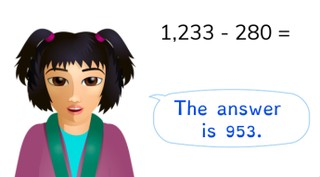Subtraction through 1,000

# Subtraction through 1,000

Students learn to subtract through 1,000.

No account needed.8,000 schools use Gynzy92,000 teachers use Gynzy1,600,000 students use Gynzy

## General

The students learn to subtract through 1,000.

## Standards

CCSS.Math.Content.4.NBT.B.4

## Learning objective

Students will be able to subtract through 1,000 to 10,000.

## Introduction

Have the students fill in the numbers to 1,000.

## Instruction

Explain that each cube always contains 1,000 blocks, every sheet 100 blocks and every row 10 blocks. This is how you can quickly count how many blocks you see in the image. Then show the problem 1,130 - 500. Count the blocks on the page together. Count how many you have in total, and then how many are taken away and how many blocks you have left over. Discuss the problem 1,230 - 350 = in the same way and have the students solve the following problem on their own. Next show the number line and explain that to solve the problem 1,133 - 571 = you must first take away the hundreds numbers, then the tens values and finally the ones values. After that, discuss the different ways to solve 1,356 - 841 = together and have the students solve the next few problems on their own. Then fill in the table together and have the students fill in the next table on their own. Finally, discuss the steps to solve a story problem. First determine what kind of problem it is and what numbers you need, then make the problem and solve it. Have the students solve the next problem on their own.

Check whether the students can subtract through 1,000 by asking the following question:
- Which method do you use to solve this problem in steps? (1,100 - 137 = ...)

## Quiz

The students are given 10 problems in which they practice with visual, abstract and story problems.They are also given a table to solve.

## Closing

Discuss once again the importance of being able to subtract through 1,000. As a closing activity, have the students click to spin the wheels and solve the resulting math problems.

## Teaching tips

Have students that have difficulty with subtracting through 1,000 first practice with splitting and filling in to 1,000.

### The online teaching platform for interactive whiteboards and displays in schools

• Save time building lessons

• Manage the classroom more efficiently

• Increase student engagement